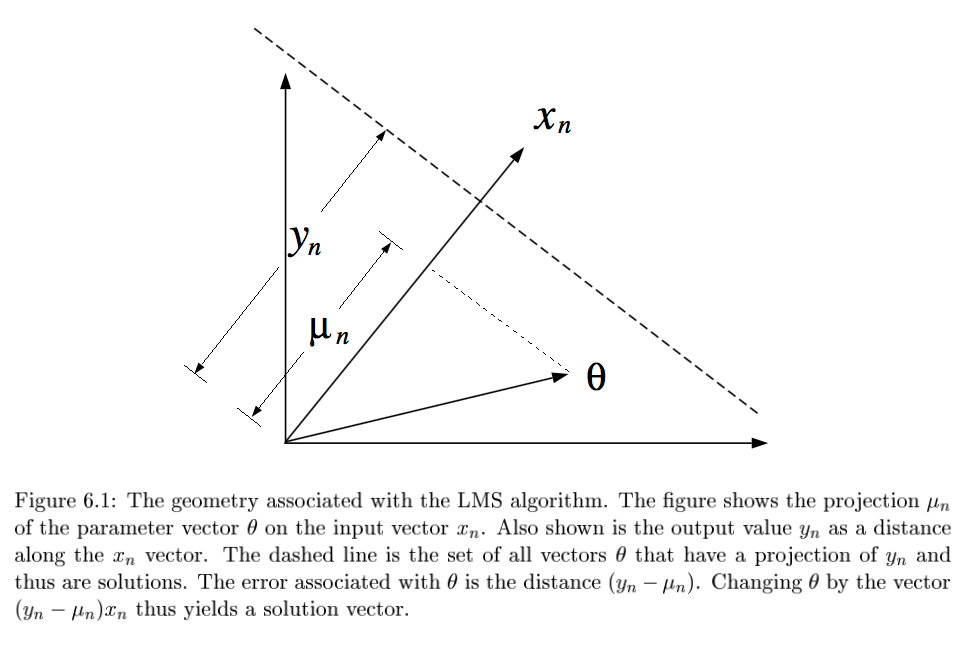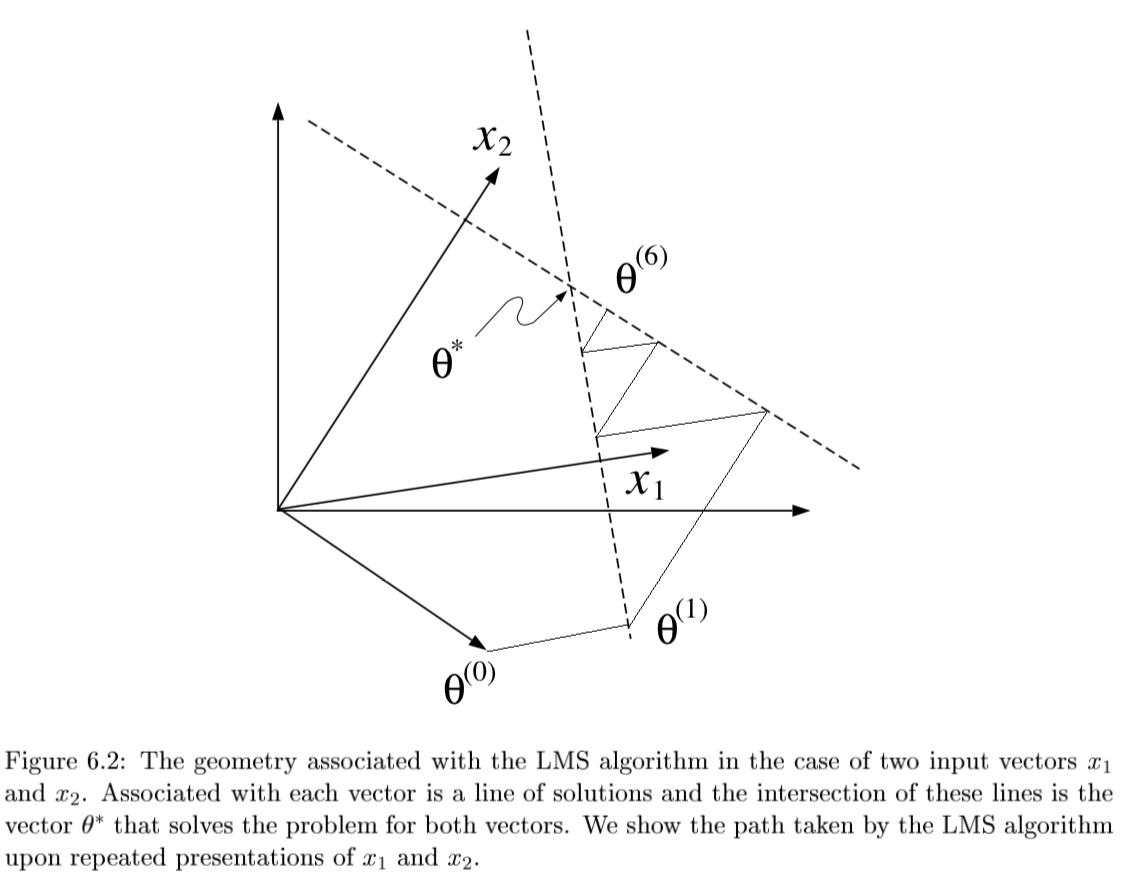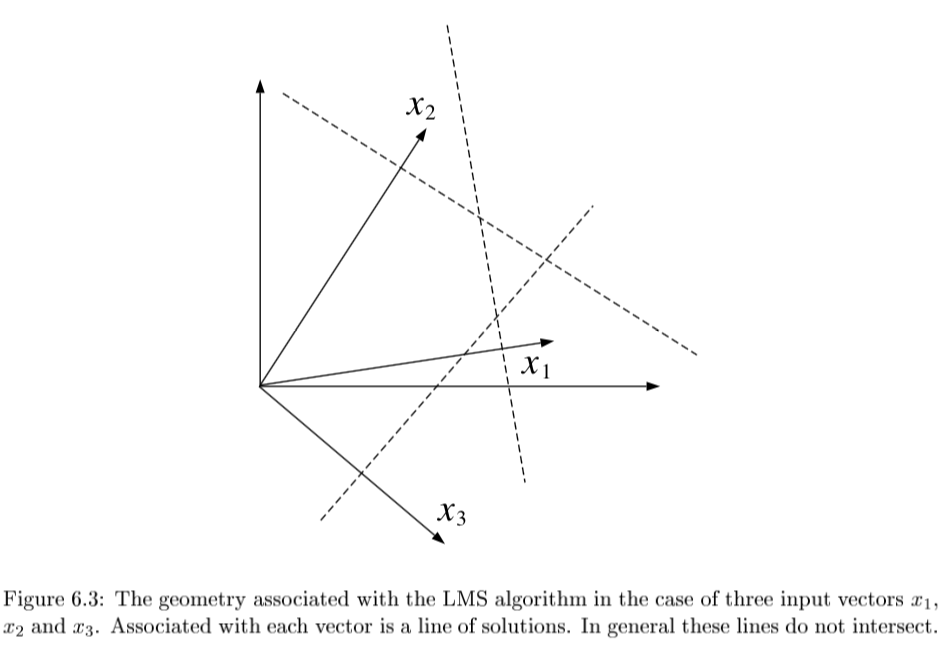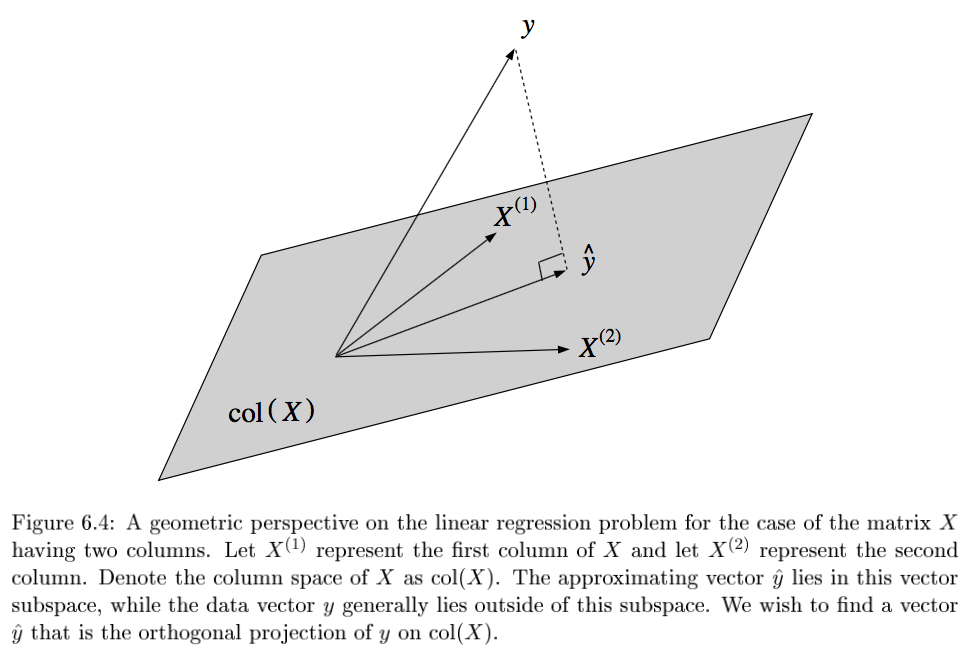linear models

• Material from “Statistical Models Theory and Practice” - David Freedman

# introduction

• $Y = X \beta + \epsilon$

type of linear regression X Y
simple univariate univariate
multiple multivariate univariate
multivariate either multivariate
generalized either error not normal
• minimize: $L(\theta) = ||Y-X\beta||_2^2 \implies \hat{\theta} = (X^TX)^{-1} X^TY$

• 2 proofs

1. set deriv and solve
2. use projection matrix H to show HY is proj of Y onto R(X)
1. the projection matrix maps the responses to the predictions: $\hat{y} = Hy$
• define projection (hat) matrix $H = X(X^TX)^{-1} X^T$
• show $||Y-X \theta||^2 \geq ||Y - HY||^2$
• key idea: subtract and add HY
• interpretation
• if feature correlated, weights aren’t stable / can’t be interpreted
• curvature inverse $(X^TX)^{-1}$ - dictates stability
• importance: weight * feature value
• LS doesn’t work when p » n because of colinearity of X columns
• assumptions
• $\epsilon \sim N(X\beta,\sigma^2)$
• homoscedasticity: $var(Y_i|X)$ is the same for all i
• opposite of heteroscedasticity
• multicollinearity - predictors highly correlated
• variance inflation factor (VIF) - measure how much the variances of the estimated regression coefficients are inflated as compared to when the predictors are not linearly related
• normal linear regression
• variance MLE $\hat{\sigma}^2 = \sum (y_i - \hat{\theta}^T x_i)^2 / n$
• in unbiased estimator, we divide by n-p
• LS has a distr. $N(\theta, \sigma^2(X^TX)^{-1})$
• linear regression model
• when n is large, LS estimator ahs approx normal distr provided that X^TX /n is approx. PSD
• weighted LS: minimize $\sum [w_i (y_i - x_i^T \theta)]^2$
• $\hat{\theta} = (X^TWX)^{-1} X^T W Y$
• heteroscedastic normal lin reg model: erorrs ~ N(0, 1/w_i)
• leverage scores - measure how much each $x_i$ influences the LS fit
• for data point i, $H_{ii}$ is the leverage score
• LAD (least absolute deviation) fit
• MLE estimator when error is Laplacian

# recent notes

## regularization

• when $(X^T X)$ isn’t invertible can’t use normal equations and gradient descent is likely unstable
• X is nxp, usually n » p and X almost always has rank p
• problems when n < p
• intuitive way to fix this problem is to reduce p by getting rid of features
• conventionally don’t regularize the intercept term
1. ridge regression (L2)
• if (X^T X) not invertible, add a small element to diagonal
• then it becomes invertible
• small lambda -> numerical solution is unstable
• proof of why it’s invertible is difficult
• argmin $\sum_i (y_i - \hat{y_i})^2+ \lambda \vert \vert \beta\vert \vert _2^2$
• equivalent to minimizing $\sum_i (y_i - \hat{y_i})^2$ s.t. $\sum_j \beta_j^2 \leq t$
• solution is $\hat{\beta_\lambda} = (X^TX+\lambda I)^{-1} X^T y$
• for small $\lambda$ numerical solution is unstable
• When $X^TX=I$, $\beta {Ridge} = \frac{1}{1+\lambda} \beta{Least Squares}$
2. lasso regression (L1)
• $\sum_i (y_i - \hat{y_i})^2+\lambda \vert \vert \beta\vert \vert _1$
• equivalent to minimizing $\sum_i (y_i - \hat{y_i})^2$ s.t. $\sum_j \vert \beta_j\vert \leq t$
• “least absolute shrinkage and selection operator”
• lasso - least absolute shrinkage and selection operator - L1
• acts in a nonlinear manner on the outcome y
• keep the same SSE loss function, but add constraint of L1 norm
• doesn’t have closed form for Beta
• because of the absolute value, gradient doesn’t exist
• can use directional derivatives
• best solver is LARS - least angle regression - if tuning parameter is chose well, will set lots of coordinates to 0 - convex functions / convex sets (like circle) are easier to solve - disadvantages
• if p>n, lasso selects at most n variables
• if pairwise correlations are very high, lasso only selects one variable
3. elastic net - hybrid of the other two
• $\beta_{Naive ENet} = \sum_i (y_i - \hat{y_i})^2+\lambda_1 \vert \vert \beta\vert \vert _1 + \lambda_2 \vert \vert \beta\vert \vert _2^2$
• l1 part generates sparse model
• l2 part encourages grouping effect, stabilizes l1 regularization path
• grouping effect - group of highly correlated features should all be selected - naive elastic net has too much shrinkage so we scale $\beta_{ENet} = (1+\lambda_2) \beta_{NaiveENet}$ - to solve, fix l2 and solve lasso

## the regression line (freedman ch 2)

• regression line
• goes through $(\bar{x}, \bar{y})$
• slope: $r s_y / s_x$
• intercept: $\bar{y} - slope \cdot \bar{x}$
• basically fits graph of averages (minimizes MSE)
• SD line
• same except slope: $sign(r) s_y / s_x$
• intercept changes accordingly
• for regression, MSE = $(1-r^2) Var(Y)$

## multiple regression (freedman ch 4)

• assumptions
1. assume $n > p$ and X has full rank (rank p - columns are linearly independent)
2. $\epsilon_i$ are iid, mean 0, variance $\sigma^2$
3. $\epsilon$ independent of $X$
• $e_i$ still orthogonal to $X$
• OLS is conditionally unbiased

• $E[\hat{\theta} | X] = \theta$
• $Cov(\hat{\theta}|X) = \sigma^2 (X^TX)^{-1}$
• $\hat{\sigma^2} = \frac{1}{n-p} \sum_i e_i^2$
• this is unbiased - just dividing by n is too small since we have minimized $e_i$ so their variance is lower than var of $\epsilon_i$
• random errors $\epsilon$
• residuals $e$
• $H = X(X^TX)^{-1} X^T$
1. e = (I-H)Y = $(I-H) \epsilon$
2. H is symmetric
3. $H^2 = H, (I-H)^2 = I-H$
4. HX = X
5. $e \perp X$
• basically H projects Y int R(X)
• $E[\hat{\sigma^2}|X] = \sigma^2$
• random errs don’t need to be normal
• variance
• $var(Y) = var(X \hat{\theta}) + var(e)$
• $var(X \hat{\theta})$ is the explained variance
• fraction of variance explained: $R^2 = var(X \hat{\theta}) / var(Y)$
• like summing squares by projecting
• if there is no intercept in a regression eq, $R^2 = ||\hat{Y}||^2 / ||Y||^2$

## BLUE

• drop assumption: $\epsilon$ independent of $X$
• instead: $E[\epsilon|X]=0, cov[\epsilon|X] = \sigma^2 I$
• can rewrite: $E[\epsilon]=0, cov[\epsilon] = \sigma^2 I$ fixing X
• Gauss-markov thm - assume linear model and assumption above: when X is fixed, OLS estimator is BLUE = best linear unbiased estimator
• has smallest variance.
• prove this

## GLS = GLM

• GLMs roughly solve the problem where outcomes are non-Gaussian
• mean is related to $w^tx$ through a link function (ex. logistic reg assumes sigmoid)
• also assume different prob distr on Y (ex. logistic reg assumes Bernoulli)
• generalized least squares regression model: instead of above assumption, use $E[\epsilon|X]=0, cov[\epsilon|X] = G, : G \in S^K_{++}$
• covariance formula changes: $cov(\hat{\theta}_{OLS}|X) = (X^TX)^{-1} X^TGX(X^TX)^{-1}$
• estimator is the same, but is no longer BLUE - can correct for this: $(G^{-1/2}Y) = (G^{-1/2}X)\theta + (G^{-1/2}\epsilon)$
• feasible GLS=Aitken estimator - use $\hat{G}$
• examples
• simple
• iteratively reweighted
• 3 assumptions can break down:
1. if $E[\epsilon|X] \neq 0$ - GLS estimator is biased
2. else if $cov(\epsilon|X) \neq G$ - GLS unbiased, but covariance formula breaks down
3. if G from data, but violates estimation procedure, estimator will be misealding estimate of cov

## path models

• path model - graphical way to represent a regression equation
• making causal inferences by regression requires a response schedule

## simultaneous equations

• simultaneous-equation models - use instrumental variables / two-stage least squares
• these techniques avoid simultaneity bias = endogeneity bias

## binary variables

• indicator variables take on the value 0 or 1
• dummy coding - matrix is singular so we drop the last indicator variable - called reference class / baseline class
• effect coding
• one vector is all -1s
• B_0 should be weighted average of the class averages
• orthogonal coding
• additive effects assume that each predictor’s effect on the response does not depend on the value of the other predictor (as long as the other one was fixed
• assume they have the same slope
• interaction effects allow the effect of one predictor on the response to depend on the values of other predictors.
• $y_i = β_0 + β_1x_{i1} + β_2x_{i2} + β_3x_{i1}x_{i2} + ε_i$

## LR with non-linear basis functions

• can have nonlinear basis functions (ex. polynomial regression)
• radial basis function - ex. kernel function (Gaussian RBF)
• $exp(-(x-r)^2 / (2 \lambda ^2))$
• non-parametric algorithm - don’t get any parameters theta; must keep data

## locally weighted LR (lowess)

• recompute model for each target point
• instead of minimizing SSE, we minimize SSE weighted by each observation’s closeness to the sample we want to query

## kernel regression

• nonparametric method

•  $\operatorname{E}(Y X=x) = \int y f(y x) dy = \int y \frac{f(x,y)}{f(x)} dy$

Using the [[kernel density estimation]] for the joint distribution ‘‘f(x,y)’’ and ‘‘f(x)’’ with a kernel ‘’’'’K’’’’’,

$\hat{f}(x,y) = \frac{1}{n}\sum_{i=1}^{n} K_h\left(x-x_i\right) K_h\left(y-y_i\right)$ $\hat{f}(x) = \frac{1}{n} \sum_{i=1}^{n} K_h\left(x-x_i\right)$

we get

 \begin{align} \operatorname{\hat E}(Y X=x) &= \int \frac{y \sum_{i=1}^{n} K_h\left(x-x_i\right) K_h\left(y-y_i\right)}{\sum_{j=1}^{n} K_h\left(x-x_j\right)} dy,\ &= \frac{\sum_{i=1}^{n} K_h\left(x-x_i\right) \int y \, K_h\left(y-y_i\right) dy}{\sum_{j=1}^{n} K_h\left(x-x_j\right)},\ &= \frac{\sum_{i=1}^{n} K_h\left(x-x_i\right) y_i}{\sum_{j=1}^{n} K_h\left(x-x_j\right)},\end{align}

## GAM = generalized additive model

• generalized additive models: assume mean output is sum of functions of individual variables (no interactions)
• learn individual functions using splines
• $g(\mu) = b + f(x_0) + f(x_1) + f(x_2) + …$
• can also add some interaction terms (e.g. $f(x_0, x_1)$)

# sums interpretation

• SST - total sum of squares - measure of total variation in response variable
• $\sum(y_i-\bar{y})^2$
• SSR - regression sum of squares - measure of variation explained by predictors
• $\sum(\hat{y_i}-\bar{y})^2$
• SSE - measure of variation not explained by predictors
• $\sum(y_i-\hat{y_i})^2$
• SST = SSR + SSE
• $R^2 = \frac{SSR}{SST}$ - coefficient of determination
• measures the proportion of variation in Y that is explained by the predictor

# geometry - J. 6

• LMS = least mean squares (p-dimensional geometries)

•• $y_n = \theta^T x_n + \epsilon_n$
• $\theta^{(t+1)}=\theta^{(t)} + \alpha (y_n - \theta^{(t)T} x_n) x_n$
•  converges if $0 < \alpha < 2/ x_n ^2$
••• if N=p and all $x^{(i)}$ are lin. indepedent, then there exists exact solution $\theta$
• solving requires finding orthogonal projection of y on column space of X (n-dimensional geometries)

•1. 3 Pfs
1. geometry - $y-X\theta^*$ must be orthogonal to columns of X: $X^T(y-X\theta)=0$
2. minimize least square cost function and differentiate
3. show HY projects Y onto col(X)
• either of these approaches yield the normal eqns: $X^TX \theta^* = X^Ty$
• SGD

• SGD converges to normal eqn
• convergence analysis: requires $0 < \rho < 2/\lambda_{max} [X^TX]$

• algebraic analysis: expand $\theta^{(t+1)}$ and take $t \to \infty$
• geometric convergence analysis: consider contours of loss function
• weighted least squares: $J(\theta)=\frac{1}{2}\sum_n w_n (y_n - \theta^T x_n)^2$

• yields $X^T WX \theta^* = X^T Wy$
• probabilistic interpretation

• $p(y|x, \theta) = \frac{1}{(2\pi\sigma^2)^{N/2}} exp \left( \frac{-1}{2\sigma^2} \sum_{n=1}^N (y_n - \theta^T x_n)^2 \right)$
• $l(\theta; x,y) = - \frac{1}{2\sigma^2} \sum_{n=1}^N (y_n - \theta^T x_n)^2$
• log-likelihood is equivalent to least-squares cost function

# likelihood calcs

## normal equation

• $L(\theta) = \frac{1}{2} \sum_{i=1}^n (\hat{y}_i-y_i)^2$
• $L(\theta) = 1/2 (X \theta - y)^T (X \theta -y)$
• $L(\theta) = 1/2 (\theta^T X^T - y^T) (X \theta -y)$
• $L(\theta) = 1/2 (\theta^T X^T X \theta - 2 \theta^T X^T y +y^T y)$
• $0=\frac{\partial L}{\partial \theta} = 2X^TX\theta - 2X^T y$
• $\theta = (X^TX)^{-1} X^Ty$

## ridge regression

• $L(\theta) = \sum_{i=1}^n (\hat{y}_i-y_i)^2+ \lambda \vert \vert \theta\vert \vert _2^2$
• $L(\theta) = (X \theta - y)^T (X \theta -y)+ \lambda \theta^T \theta$
• $L(\theta) = \theta^T X^T X \theta - 2 \theta^T X^T y +y^T y + \lambda \theta^T \theta$
• $0=\frac{\partial L}{\partial \theta} = 2X^TX\theta - 2X^T y+2\lambda \theta$
• $\theta = (X^TX+\lambda I)^{-1} X^T y$